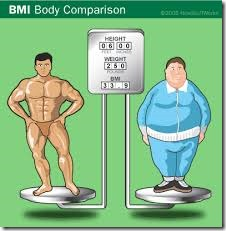# Am I Over Weight? BMI and BMI Prime:Do you want to know whether you are overweight or not?
You must keep in mind there is a scientific method to know whether you are overweight or underweight. Many people are concerned about their weight and sometimes many individuals especially girls, start thinking that they are over-weight but actually they aren’t. Such girls stop eating and become very thin and weak. This condition is called anorexia nervosa.
The scientific method used to find out about weight is called the Body-Mass Index.

### Factors on which Weight Depends:

Body Mass Index depends on two factors:
• Body Mass
• The height of the body.

1. Body Mass:
Mass is the weight of the body. (Mass and weight are mostly considered same biologically but are physically different quantities) The Mass of the body is measured in Kilograms or pounds. Most of the Asian countries use Kilogram as a unit to measure mass while most of the western countries use pound to measure mass.

2. The height of Body:
Only mass can’t be used to declare someone over or underweight. One can’t tell another person’s weight status without knowing his height. For example, if your height is 5 feet and your weight is 70 kg and there is another person whose height is 6 ft and his weight is 70 kg then you are overweight but the other person has normal weight as his height is more.

### How to calculate Body Mass Index:

Let us consider the above example. We will calculate the BMI of a person (PERSON A) whose height is 5 feet and his weight is 70 kg.
the formula of BMI is given below
BMI =       weight in kilogram
[ height in meters ]2
To calculate BMI, we use height in meter and weight in kilograms. So we have to convert 5 feet into meters.
1 feet = 12 inches
5 feet = 12 x 5 = 60 inches
39 inches = 1 meter
60 inches = 60/39 meters
60 inches = 1.53 meters.
look at the formula, the height is used in the square. So we will take square the height.
1.53m x 1.53m = 2.35m2
We already have weight in kilograms. (if you have measure weight in pounds then multiply the weight in pounds with 0.45 to convert it into kilograms)
Now use the formula.
BMI = 70/2.35 = 29.78

So the BMI of this person is 29.78.

let us consider the BMI of another person with a height of 6 feet and a weight of 70 kg (PERSON B)
6 feet = 72 inches
72 inches = 1.84 meters
Now by using the formula, the BMI will be:
BMI = 70/3.39 = 20.64

Now you have noticed a clear difference in BMI of two persons with different heights.

### Interpretation of BMI result:

 Category BMI range – kg/m2 BMI Prime Comments: Very severely underweight <15 <0.60 Consult Doctor Severely underweight 15.0 to 16.0 0.60 to 0.64 Consult Doctor Underweight 16.0 to 18.5 f0.64 to 0.74 Consult Doctor Normal (healthy weight) 18.5 to 25 0.74 to 1.0 Eat healthily Overweight 25 to 30 1.0 to 1.2 Do Regular Exercise and Modify your diet Obese Class I (Moderately obese) 30 to 35 1.2 to 1.4 Consult Doctor Obese Class II (Severely obese) 35 to 40 1.4 to 1.6 Consult Doctor Obese Class III (Very severely obese) >40 >1.6 Consult Doctor

Now from the above result, we can say that PERSON A is Overweight but not obese. He should do regular exercise, he should modify his dietary habits. He should add vegetable and fruits in his diet and skip beef, fried foods and foods high in fat and cholesterol. PERSON B has a BMI of 20.6 and it is in a healthy range.

### BMI Prime:

BMI prime is a modification of BMI. To calculate the BMI prime, divide your BMI by 25 (upper limit normal healthy BMI). Individuals with BMI prime ranges from “0.74 to 1”  are considered normal and healthy. Individuals having BMI prime of less than 0.74 are considered underweight and those having BMI prime more than 1 are considered overweight.